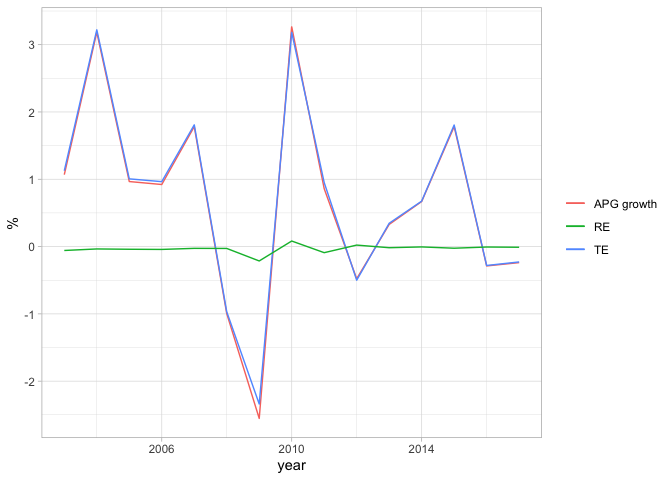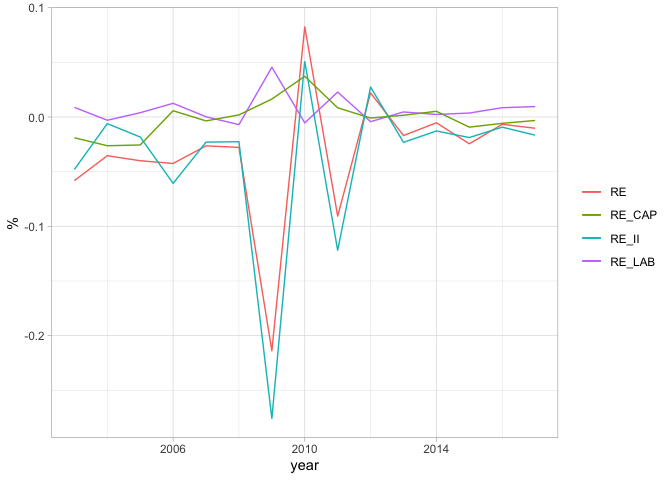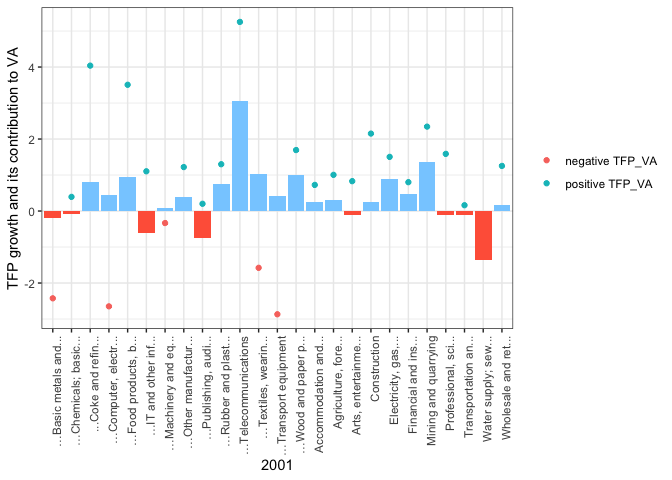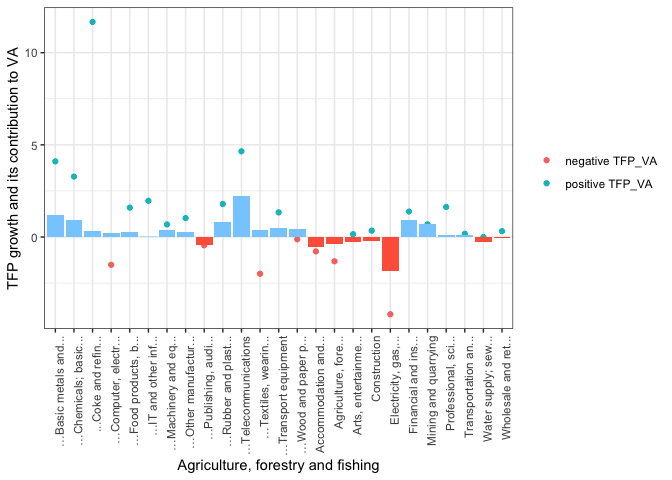# Aggregate Productivity Growth Decomposition

The theory is derived from the original paper: Measuring aggregate productivity growth using plant-level data by Petrin & Levinsohn (2013). I learned about this model from the Applied Macroeconomics course by Professor Suzuki course.

## What is APG?

Conventionally, Solow growth accounting provides a tractable framework for us to see the contribution of capital, labor, and TFP (or Solow residual). However, the problem with this method is that it assumes the perfect efficient allocation of inputs. That is, there is no distortion in the economy, capital and labor are allocated perfectly so that the sole contribution to TFP is simply technological progress. In the long-term, this assumption may be reasonable, however, in the short term, it becomes too restrictive. For instance, the Lehman shock in 2008 may have caused large frictions in the financial sector, causing capital and labor to move to other sectors, which could have improved aggregate productivity. The simple Solow accounting cannot capture this effect.

Petrin & Levinsohn (2013) provide a method to further decompose Solow residual growth into 2 portions: TE (technological efficiency, a.k.a technological progress) and RE (reallocation efficiency of inputs). The inputs include capital, labor, and intermediate goods. The Solow residual is now referred to as APG (aggregate productivity growth). We decompose TE and RE and then use the EUKLEMS sector-specific micro-level data to quantify such effects and examine how much TE and RE contribute to APG.

The economy in this growth accounting exercise is Belgium, but the model can be extended to any country with sufficient data similar to EUKLEMS.

## Production of a representative firm

The gross output production function for firm i:

$Q_i = Q_i (L_i, X_i, \omega_i)$

where:

$Q_i = \text{firm i’s output}$

$L_i = \text{firm i’s primary inputs} = {L_{ik}} = (L_{i1}, L_{i2}, …, L_{ik})$ => firm i uses inputs from sector k. Primary inputs include labor and capital

$X_i = \text{firm i’s intermediate inputs} = {X_{ij}} = (X_{i1}, …, X{ij})$ => firm i uses output of firm j

$\omega_i = \text{firm i’s technical efficiency}$

Measure the change in $Q_i$:

$d Q_i = \frac{\partial Q_i}{\partial L_i}d L_i + \frac{\partial Q_i}{\partial X_i}d X_i + \frac{\partial Q_i}{\partial \omega_i}d \omega_i$

Notice that:

$d L_i = \frac{\partial L_i}{\partial L_k}dL_{ik}$, $d X_i = \frac{\partial X_i}{\partial X_j}d X_{ij}$

so:

$d Q_i = \frac{\partial Q_i}{\partial L_k}d L_{ik} + \frac{\partial Q_i}{\partial X_j}d X_{ij} + \frac{\partial Q_i}{\partial \omega_i}d\omega_i$

## Consumers

Final production that goes to consumers should be:

$Y_i = Q_i - \sum_j X_{ji}$

where:

$Q_i = \text{firm i’s total production}$

$\sum_j X_{ji} = \text{total output from firm i that is used as intermediate goods}$

$\sum_i Y_i$ should be the GDP (production goes to consumers, after eliminating all intermediate goods)

so:

$dY_i = dQ_i - \sum_j dX_{ji}$

which means:

$\Rightarrow d Y_i = \frac{\partial Q_i}{\partial L_k}d L_{ik} + \frac{\partial Q_i}{\partial X_j}d X_{ij} + \frac{\partial Q_i}{\partial \omega_i}d\omega_i - \sum_j dX_{ji}$

## Determination of APG

By definition, APG measures the change in aggregate final demand and the change in aggregate inputs.

We want to know how much the economy gains from such changes in input allocation.

$APG \equiv \color{red}{\sum_i P_i \ dY_i} - \color{blue}{\sum_i\sum_k W_{ik} \ dL_{ik}}$

where the first term is the gain from output itself (namely by technological innovations) minus the second term, which is the “gain” from the changes in the cost of inputs, which are labor and intermediate goods.

Intermediate steps:

\begin{aligned} &\color{red}{\sum_i P_i \ dY_i} = \sum_i P_i \left(\frac{\partial Q_i}{\partial L_k}d L_{ik} + \frac{\partial Q_i}{\partial X_j}d X_{ij} + \frac{\partial Q_i}{\partial \omega_i}d\omega_i - \sum_j dX_{ji}\right) \\ &= \sum_i P_i\frac{\partial Q_i}{\partial \omega_i}d\omega_i + \sum_i\sum_k P_i\frac{\partial Q_i}{\partial L_k}dL_{ik} + \sum_i\sum_jP_i\frac{\partial Q_i}{\partial X_j}dX_{ij} - \sum_i P_i\left(\sum_j dX_{ji}\right) \\ &= \sum_i P_i\frac{\partial Q_i}{\partial \omega_i}d\omega_i + \sum_i\sum_k P_i\frac{\partial Q_i}{\partial L_k}dL_{ik} + \sum_i\sum_jP_i\frac{\partial Q_i}{\partial X_j}dX_{ij} - \sum_i\sum_j P_j dX_{ij} \end{aligned}

(Note (*) : The last terms is obtained because $\sum_i\sum_j P_i X_{ij} = \sum_i\sum_j P_j X_{ji}$)

so we rewrite the APG formula as follows.

\begin{aligned} &APG = \color{red}{\sum_i P_i\frac{\partial Q_i}{\partial \omega_i}d\omega_i + \sum_i\sum_k P_i\frac{\partial Q_i}{\partial L_k}dL_{ik} + \sum_i\sum_j(P_i\frac{\partial Q_i}{\partial X_j} - P_j)\ dX_{ij}} - \color{blue}{\sum_i\sum_k W_{ik} \ dL_{ik}} \\ &= \color{red}{\sum_i P_i\frac{\partial Q_i}{\partial \omega_i}d\omega_i} + \color{blue}{\sum_i\sum_k ( P_i\frac{\partial Q_i}{\partial L_k} - W_{ik})\ dL_{ik}} + \color{green}{\sum_i\sum_j(P_i\frac{\partial Q_i}{\partial X_j} - P_j)\ dX_{ij}} \end{aligned}

Meaning:

$\color{red}{red}$: gain from technical efficiency changes (TE)

$\color{blue}{blue}$: gain from reallocation of pirimary nput factors such as Labor and Capital (RE_lab, RE_cap)

$\color{green}{green}$: gain from reallocation of intermediate goods (RE_ii)

$\Rightarrow$ We can see that the change in final demand (output) comes from 3 sources: tech growth, RE in inputs, and RE of intermediate goods.

This is one of the most important equations to decompose APG. The next thing we want to do is to measure and decompose its rate of change $g_{APG}$.

## The rate of change of APG

To find the formula for $g_{APG}$, we introduce the term VA.

Basically, VA is the additional value a firm i adds to the value of intermediate goods. When firm i buys intermediate goods to produce stuff and then sells it, the difference is the value-added. By definition:

${VA}_i \equiv P_iQ_i - \sum_j P_j X_{ij} = P_i(Y_i + \sum_j X_{ji}) - \sum_j P_j X_{ij}$

$\sum_i VA_i = \sum_i P_i Y_i + \sum_i\sum_jP_iX_{ji} - \sum_i\sum_jP_jX_{ij} = \sum_i P_iY_i$ (the last 2 terms cancel out, see Note(*))

Since Y is the final output, $\sum_i P_i Y_i$ is equivalent to the nominal GDP.

Furthermore, since APG measures the total changes in production, i.e., APG by itself indicates the change from the baseline output (nominal GDP $\equiv \sum_i VA_i$).

### APG Growth

The Aggregate Productivity Growth is defined as:

$g_{APG} = \frac{APG}{\sum_i VA_i}$

There are 2 ways to estimate $g_{APG}$

#### (1) Use the Solow residual as a proxy

Decomposition of $g_{APG}$ gives:

\begin{aligned} &g_{APG} = \frac{APG}{\sum_i VA_i} = \frac{1}{\sum_i VA_i} \left(\sum_i P_i d Y_i - \sum_i\sum_k W_{ik} dL_{ik}\right) \\ &= \sum_i \frac{P_i Y_i}{\sum_i VA_i}\frac{dY_i}{Y_i} - \sum_k\sum_i \frac{W_{ik}L_{ik}}{\sum_i VA_i}\frac{d L_{ik}}{L_{ik}} \end{aligned}

This is actually the Solow residual:

$\frac{\dot{Y}}{Y} - \alpha_K\frac{\dot{K}}{K} - \alpha_L\frac{\dot{L}}{L}$

Proof:

$g_{APG} = \color{red}{\sum_i \frac{P_i Y_i}{\sum_i VA_i}\frac{dY_i}{Y_i}} - \color{blue}{\sum_k\sum_i \frac{W_{ik}L_{ik}}{\sum_i VA_i}\frac{d L_{ik}}{L_{ik}}}$

since $\sum_i VA_i = \sum_i P_iY_i$ so

$\color{red}{\sum_i \frac{P_i Y_i}{\sum_i VA_i}\frac{dY_i}{Y_i}} = \sum_i \frac{dY_i}{Y_i} = \frac{dY}{Y}$

This is the total change in GDP.

Furthermore:

$\color{blue}{\sum_k\sum_i \frac{W_{ik}L_{ik}}{\sum_i VA_i}\frac{d L_{ik}}{L_{ik}}} = \sum_k \left( \frac{\sum_i (W_{ik}L_{ik})}{\sum_i VA_i}\right)\sum_i\left(\frac{W_{ik}L_{ik}}{(W_{ik}L_{ik})}\frac{dL_{ik}}{L_{ik}}\right)$

The first term $\sum_k$ is the factor income share a la Solow $\equiv \alpha_k$

The second term $\sum_i$ is the total change in input factors

Thus, $g_{APG} = \frac{dY}{Y} - \sum_k \alpha_k \frac{dL_k}{L_k}$

Comment: This estimation might be overestimated because it includes several sectors that are not productive or of foreign entities.

#### (2) Use VA

Define the change in $VA_i$ as:

$dVA_i \equiv P_i\ dQ_i - \sum_j P_j \ dX_{ij}$

Aggregation gives:

$\sum_i dVA_i = \sum_i P_i dQ_i - \sum_i\sum_j P_j dX_{ij}$

Plugging it into the following equation gives:

\begin{aligned} &APG = \sum_i P_i dY_i - \sum\sum W_{ik}dL_{ik} = \sum_i P_i dQ_i - \sum\sum P_j dX_{ij} - \sum\sum W_{ik}dL_{ik} \\ &\equiv \sum_i dVA_i - \sum\sum W_{ik}dL_{ik} \end{aligned}

Using the definition of $g_{APG}$ , we have:

$g_{APG} = \frac{APG}{\sum_i VA_i} = \frac{\sum_i d VA_i - \sum\sum W_{ik}dL_{ik}}{\sum_i VA_i}$

Note that, by taking logs and differentiating wrt time, we have:

Let $y = \ln(VA)$

\begin{aligned} &\Rightarrow \frac{dy}{dt} = \frac{dy}{d\ VA}\frac{dVA}{dt} = \frac{1}{VA}\frac{dVA}{dt} \\ &\Rightarrow \frac{dVA_i}{dt} = VA_i \frac{dy}{dt} \\ &\Rightarrow d\ VA_i = VA_i d \ln(VA_i) \end{aligned} Likewise for the other term:

$\frac{dL_{ik}}{dt} = W_{ik}L_{ik} \frac{d\ln(L_{ik})}{dt} \Rightarrow W_{ik}dL_{ik} = \frac{W_{ik}L_{ik}}{VA_i} \ VA_i d\ln(L_{ik})$

So $g_{APG}$ can be rewritten as:

\begin{aligned} &g_{APG} = \frac{1}{\sum VA_i} \left(\sum_i dVA_i- \sum\sum W_{ik} dL_{ik}\right) \\ &\Rightarrow g_{APG} = \frac{1}{\sum VA_i} \left(VA_i \sum_i d \ln(VA_i) - VA_i \frac{W_{ik}L_{ik}}{VA_i} d\ln(L_{ik})\right) \\ &\Rightarrow \color{red}{g_{APG}= \sum_i D_i^\nu d \ln(VA_i) - \sum_i D_i^\nu \sum_k s^\nu_{ik} d \ln(L_{ik})} \end{aligned}

where: $D_i^\nu = \frac{VA_i}{\sum_{i} VA_i}$, also known as the Domar Weight, $s_{ik}^\nu = \frac{W_{ik}L_{ik}}{VA_i}$

#### Referring to EUKLEMS dataset

$VA_i$: dataNA / VA (GVA, current prices)

$W_{ik}L_{ik}$: dataGA / LAB (Labor compensation) & CAP (capital compensation)

$\ln(L)$ : take logs of dataGA / LAB_QI (labor services) & CAP_QI (capital services)

$\ln(VA_i)$: take logs of dataNA / VA_Q

Thus, from the formula in red, we can estimate APG growth.

### Decomposition of $g_{APG}$

Recall:

$APG = \color{red}{\sum_i P_i\frac{\partial Q_i}{\partial \omega_i}d\omega_i} + \color{blue}{\sum_i\sum_k ( P_i\frac{\partial Q_i}{\partial L_k} - W_{ik})\ dL_{ik}} + \color{green}{\sum_i\sum_j(P_i\frac{\partial Q_i}{\partial X_j} - P_j)\ dX_{ij}}$

Using the same technique as before, it is not hard to derive that:

$\sum_i P_i\frac{\partial Q_i}{\partial \omega_i}d\omega_i = \sum_i P_i \frac{\partial Q_i}{\partial \omega_i}\omega_i d \ln(\omega_i) = \sum_i P_iQ_i\frac{\partial Q_i}{\partial \omega_i}\frac{\omega_i}{Q_i} d \ln(\omega_i)$

$\sum_i\sum_k ( P_i\frac{\partial Q_i}{\partial L_k} - W_{ik})\ dL_{ik} = \sum_i P_iQ_i\sum_k\left( \frac{\partial Q_i}{\partial L_{ik}} \frac{L_{ik}}{Q_i} - \frac{W_{ik}L_{ik}}{P_iQ_i}\right) d\ln(L_{ik})$

$\sum_i\sum_k ( P_i\frac{\partial Q_i}{\partial X_j} - P_{j})\ dX_{ij} = \sum_i P_iQ_i\sum_j \left( \frac{\partial Q_i}{\partial X_{ij}} \frac{X_{ij}}{Q_i} - \frac{P_{ij}X_{ij}}{P_iQ_i}\right) d\ln(X_{ij})$

Using the formula of $g_{APG} = \frac{APG}{\sum_i VA_i}$ , we get:

$g_{APG} = \frac{APG}{\sum VA_i} = \color{blue}{\sum_i D_i \sum_k (\beta_{ik} - s_{ik})d \ln(L_{ik})} + \color{green}{\sum_i D_i \sum_j (\beta_{ij} - s_{ij})d \ln(X_{ij})} + \color{red}{\sum_i D_i \beta_w d\ln(\omega_i)}$

where $\frac{P_iQ_i}{\sum_i VA_i} \equiv D_i$ <- Gross Output Domar Weight

$\beta$ term is the Elasticity of output wrt input $\beta_{ik} = \frac{\partial Q_i}{\partial L_{ik}}\frac{L_{ik}}{Q_i}$, $\beta_{ij} = \frac{\partial Q_i}{\partial X_{ij}}\frac{X_{ij}}{Q_i}$

$s$ as factor income share: $s_{ik} = \frac{W_{ik}L_{ik}}{P_iQ_i}, s_{ij} = \frac{P_{ij}X_{ij}}{P_iQ_i}$

From here, Reallocation term (blue and green) can be dervied explicitly while the red term is derived as the residual

#### Referring to the EUKLEMS dataset

$P_iQ_i$ : dataNA / GO (Gross Output, current prices

$W_{ik}L_{ik}$: dataGA/ LAB & CAP (labor and capital compensation) -> for calculation of $s_{ik}$

$P_{ij}X_{ij}$: dataNA / II (intermediate inputs) -> for calculation of $s_{ij}$

$d \ln{L_{ik}}$ : the change in the logs of real inputs (take logs of dataGA/ LAB_QI, CAP_QI )

$d \ln(X_{ij})$: the change in the logs of real inputs (take logs of dataNA/ II_Q )

Estimation for $\beta$ is trickier. In the long run, elasticity $\beta$ should converge towards factor income share $s$, but not neccessarily in the short-run. When we examine its dynamics over long period of time, taking the average of factor income share can also be a good approximation for $\beta$ during that period.

## Period and Demonstrative Graphs

### Data

library(knitr)
library(ggplot2)
library(dplyr)
library(plotly)
#---- changing codes here ---- #
#--change the country here
country_name <- c('Belgium')
#--change penn world data timeframe
pwt_from = 1959 #recommend from 1959 for evenly spaced
pwt_to = 2019 #recommend the latest data
pwt_interval = 10 #declare interval
#--change APG decomposition timeframe
apg_year_from = 2001 #the earliest data available, check by opening the excel file
apg_year_to = 2017 #check by opening the excel file
apg_year_interval_small = 4 #small interval for APG decomposition
apg_year_interval_big = 8 #big interval for TFP's industry decomposition
#--select variables and industries --#
variables = c("LAB","CAP","LAB_QI","CAP_QI","VA","GO","II_Q","II","VA_Q","GO_Q","EMP")
non_market_industries = c('L','O','P','Q','T','U') #edit to empty list to include
agg_industries = c("A","B","C","D","E","F","G","H","I","J","K","M_N","R_S") #grouped and aggregate industries
nagg_industries = c("A","B", "C10-C12","C13-C15","C16-C18","C19","C20_C21","C22_C23","C24_C25","C26_C27","C28","C29_C30","C31-C33","D","E","F","G","H","I","J58-J60","J61","J62_J63","K","M_N","R_S") #uncombined non aggregate industries
#for Solow:
#for APG


The development of the Belgian economy can be divided into the following sub-periods:

• 1960 - 1980: Growth was fostered by foreign investment, notably from the US
• 1980 - 1990: Following the Oil Shock in 1979 was a recession in 80-82. This period also marks the shift in the regional economic structure where Flanders gradually took Wallonia’s place as the economic center.
• 1990 - 1999: Economy headed towards more export-centric and further European integration.
• 1999 - 2009: The economy was fostered by regional dynamics and ICT’s advancement.
• 2009 - 2019: The economy is in recovering phase after the GFC.

We use EUKLEMS data here (available from 1995) Notice that both NA (national account) and GA (growth account) are necessary. Then create a subset data for Belgium.

#---- Load data ---- #
euklems_NA <- subset(dataNA, country == country_name_apg)
euklems_GA <- subset(dataGA, country == country_name_apg)


### The Idea

1. We import important variables from the subset.
2. Calculate the Domar weights (VA and GO). From here, we can calculate $\beta$
3. $\beta$ Estimation
• Method 1: Discrete time approximation of RE term
$\int_t^{T} g_{APG}(RE) \approx \sum_i\sum_k \bar{D_{it}} (\bar{\beta}*{ik,t} - \bar{s*{ik,t}})\ d\ln(L_{ik,t}) + \sum_i\sum_j\bar{D_{it}} (\bar{\beta}*{ij,t} - \bar{s*{ij,t}})\ d\ln(X_{ij,t})$

where: $\bar{x_t} \equiv \frac{x_t + x_T}{2}$ the moving average between 2 periods

$\bar{\beta} = \frac{\sum_{t=1}^T s_t}{T}$ : elasticity as the average sum from the beginning of time until point T in time

• Method 2: Assume a functional form for productivity and estimate

For example, assume a Cobb-Douglas PF:

$Q_i = \omega L^{\beta_1}K^{\beta_2}X^{\beta_3}$

take logs and perform OLS estimation:

$\ln Q_i = \hat{\beta_0} + \hat{\beta_1} \ln(L) + \hat{\beta_2} \ln(K) + \hat{\beta_3} \ln(X) + \ln(\omega)$

PF is not necessarily CRS ($\sum \beta \ \text{can} \ne 1$).

Since it’s hard to control for the endogeneity in OLS estimation of $\beta$, we use method 1. Method can be useful for estimating TFP for each industry.

1. Divide the time periods then calculate the difference & weighted moving average
2. Perform growth accounting

### Code (hidden)

The flow:

#### Aggregate Industries

1. Subset the data

2. Calculate the Domar weights and logs of variables

3. Calculate beta

#### Aggregate industry APG | PERIODS

1. Setup the time periods and calculate dx/dt

2. Peform APG decomposition

Results:

year          g_APG_annual   TE_annual   RE_annual   RE_LAB_annual   RE_CAP_annual   RE_II_annual
2001-2005           1.6861      1.6985     -0.0130          0.0022          0.0020        -0.0172
2005-2009          -0.2286     -0.1944     -0.0341          0.0092          0.0110        -0.0543
2009-2013           0.9354      0.8894      0.0473         -0.0031          0.0160         0.0344
2013-2017           0.4626      0.4596      0.0030         -0.0010         -0.0002         0.0042


Comment:

Growth was high during the pre-GFC era.

GFC hit Belgium pretty hard but Belgium recovered fast as well.

#### Aggregate industry APG | YOY

1. Calculate the changes

2. Perform APG decomposition

Results

year   g_APG_annual   TE_annual   RE_annual   RE_LAB_annual   RE_CAP_annual   RE_II_annual
2003         1.0684      1.1266     -0.0582          0.0088         -0.0190        -0.0480
2004         3.1851      3.2205     -0.0354         -0.0030         -0.0263        -0.0061
2005         0.9661      1.0061     -0.0400          0.0039         -0.0256        -0.0183
2006         0.9220      0.9645     -0.0425          0.0125          0.0057        -0.0607
2007         1.7819      1.8083     -0.0264          0.0002         -0.0036        -0.0230
2008        -0.9968     -0.9690     -0.0278         -0.0070          0.0019        -0.0226
2009        -2.5537     -2.3398     -0.2138          0.0455          0.0164        -0.2757
2010         3.2641      3.1817      0.0824         -0.0054          0.0372         0.0506
2011         0.8595      0.9502     -0.0907          0.0228          0.0084        -0.1219
2012        -0.4780     -0.5000      0.0220         -0.0043         -0.0010         0.0274
2013         0.3290      0.3460     -0.0170          0.0046          0.0016        -0.0232
2014         0.6695      0.6748     -0.0053          0.0023          0.0052        -0.0128
2015         1.7818      1.8062     -0.0245          0.0036         -0.0093        -0.0188
2016        -0.2878     -0.2811     -0.0067          0.0085         -0.0058        -0.0093
2017        -0.2386     -0.2283     -0.0103          0.0095         -0.0032        -0.0166


The plot of APG growth, TE and RE:RE is indeed stable, meaning the economy seems to be in the frictionless state/ efficient throughout most of the time.

Gains from reallocation seem to be more pronounced following negative productivity shocks. In this case, RE was positive after the GFC, and mostly from the capital and intermediate inputs. Belgium’s economy performed pretty well after the GFC despite the European Debt Crisis during (2008-2012).

However, as the ECB was pursuing QE policy (APP) very strongly from 2016 until the end of 2018 to avoid devaluation of the Euro, the price of the Euro has steadily increased. As the result, [Belgium’s export was modest] during from 2015 to 2017 and lower than the previous years, which may have contributed to significantly slower growth during this period.

The plot of RE terms only:The movement of in labor is always positive, while CAP and II are more disruptive.

Most of the movements in RE are the result of II. Perhaps, as manufacturing and export of finished products are what Belgium is good for. Negative shift mainly due to international disruptions in the supply chain. The swift positive reallocation of II shows that domestic II is quite strong, but maybe not as competitive as imported.

#### Industry’s TFP Growth vs contribution to VA growth

Period 1:Period 2:Decling industries: computer parts, textiles, transportation, publishing, wood and paper, energy.

Raising industries: Professional, Chemicals

The share of employment in manufacturing has been declining, albeit its GO Domar weight remains roughly unchanged. A larger part of the job pool is now reserved for the Professional and scientific sector, indicating a larger role of knowledge in economic growth. The share of Domar weight of this sector to Gross Output (GO) has been also increasing over time.

Written on June 6, 2021